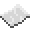# math.pow

Return x raised to the yth power. This is equivalent to the operator `^`.ExamplePrint the n-th power of two
Read a line from the user, then print two raised to that number.
Code
```<nowiki>
</nowiki>
```
Output Depends on what the user wrote. For instance, if they were to enter `5`, the value printed would be `32`.

math.pow
Function
Syntax
math.pow(
• x : number
• y : number
)

Returns number
API math
Source Lua (source)